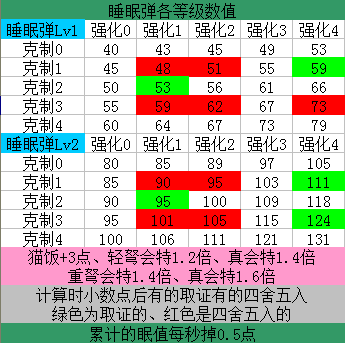注册

# 《怪物猎人：世界》各种武器异常属性会心倍率测试

• 来源：百度贴吧
• 作者：jack_7826
• 编辑：月色如画
0− 测试过程 ...

57*0.7=39.9=40，与实际相符

56*0.7=39.2=39，与实际相符

57*0.69=59.33，四舍五入不足40

58*1.2=69.6

57*1.2=68.4

58*1.19=69.02，排除1.19

57*1.22=69.54,排除1.22

1、先计算会心四舍五入后，计算化合衣

47*1.21=56.87=57

57*0.7=39.9=40,与实际不符

2、先计算化合衣四舍五入后，计算会心

47*0.7=32.9=33

33*1.21=39.93=40,与实际不符

3、一起计算然后四舍五入

47*1.21*0.7=39.809=40，与实际不符

1、还是武器面板470，穿化合衣、技能属性异常会心，砍骨锤龙第一刀不可以爆破

47*0.7=32.9=33，33*1.2=39.6=40，与实际不符

2、武器面板710，穿化合衣、技能真属性异常会心，砍毒妖鸟第一刀可以爆破

71*1.4=99.4=99，99*0.7=69.3=69，与实际不符

71*1.4*0.7=69.58=70，与实际相符全新动作 大剑 太刀 飞翔爪（新） 片手剑 双剑 狩猎笛 大锤 长枪 铳枪 斩斧 盾斧 操虫棍 弓 轻弩 重弩 全新版本 预购特典 各版本内容 新登场怪物 大师等级 新地图:永霜冻土 杂志情报

 基础入门 联机系统 手柄按键操作 骑乘系统 武器锐利度 炼金系统 据点设施 调和表 名词术语 装备特性 剧情任务 组队须知 必学小知识 高手进阶 猫饭技能效果 PC版平民向盾斧配装 采集流配装 PC太刀连招 随从猫技能选择 客制强化全效果 武器属性值上限 操虫棍空中流玩法 投射器使用技巧 狩猎笛全buff 狩猎笛音色 资深猎人必备知识 资深玩法 速刷历战痕迹 历战恐暴龙 历战双爆鳞龙 弓箭历战炎妃龙 双刀历战麒麟 历战钢龙 包过灭尽龙打法 斗技大会打法 操虫棍猎虫解读 客制强化效果 太刀速刷灭尽 极贝爷打法

MOD专区

 《怪物猎人：世界》实用MOD推荐 MOD大全 特效MOD大全 实用工具大全 修改器大全 武器MOD大全 服装MOD大全8.4

• 类型：动作游戏
• 发行：Capcom
• 发售：2018-08-10（PC）
• 开发：Capcom
• 语言：简中
• 平台：PC PS4 XBOXONE
• 标签：合作狩猎3DM自运营游戏推荐 更多+
•三国群英传

•神座

•开始游戏 进入官网•开始游戏 进入官网